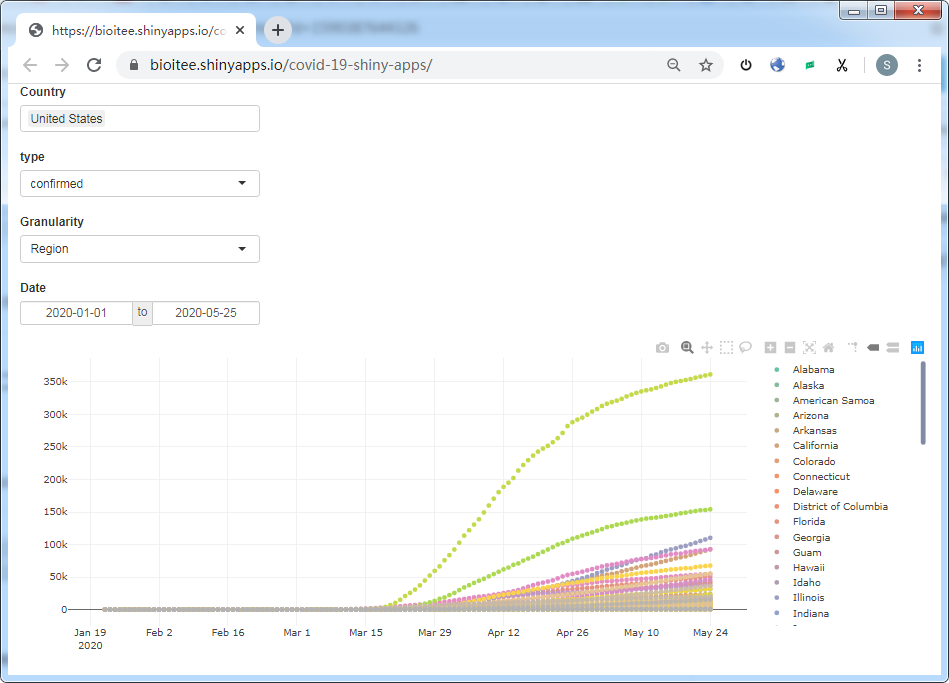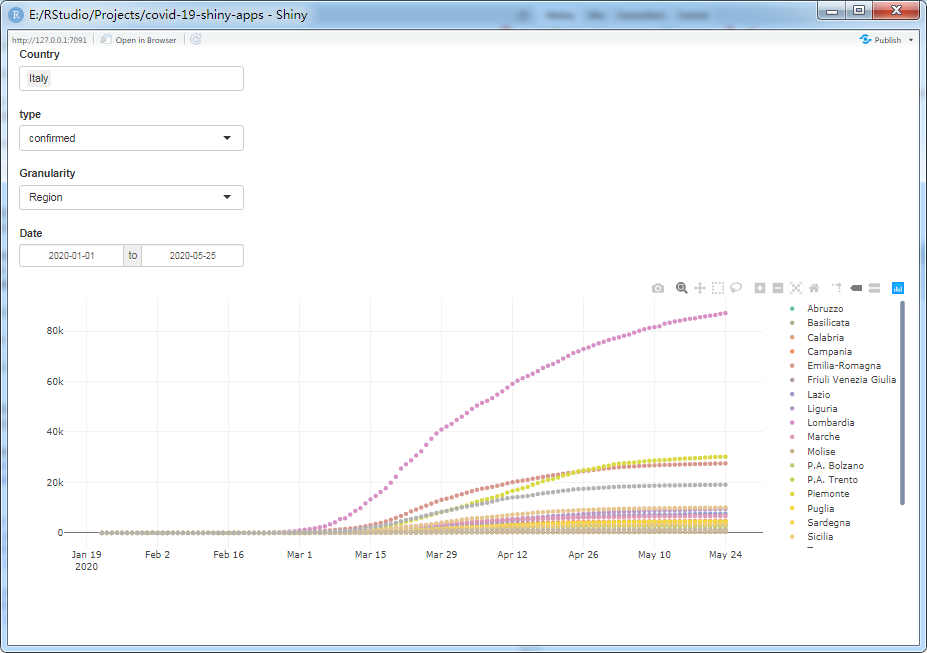The goal of COVID-19 Data Hub is to provide the research community with a unified data hub by collecting worldwide fine-grained case data, merged with exogenous variables helpful for a better understanding of COVID-19.

```library(shiny)
library(plotly)
library(COVID19)```

## COVID19

COVID19 的 R 包通过 `COVID19()` 函数提供了与新型冠状病毒肺炎数据中心的无缝集成。关于这个函数的更多参数说明，我们可以通过 `?covid19` 进行查看。下面我们将使用：

• `country`：vector of country names or ISO codes. 国家名称或国际标准化组织代码的矢量。
• `level`：granularity level; data by (1) country, (2) region, (3) city. 粒度级别；按(1)国家、(2)地区、(3)城市分列的数据。
• `start`：the start date of the period of interest. 感兴趣的开始日期。
• `end`： the end date of the period of interest. 感兴趣的结束日期。

## 定义用户界面

• country：the country name. Note that the options are automatically populated using the `covid19()` function. 国家名称。请注意，选项是使用 covid19() 函数
• type：the metric to use. One of `c("confirmed", "tests", "recovered", "deaths")`, but many others are avaibale. See here for the full list. 使用的度量标准，可以是 `c("confirmed", "tests", "recovered", "deaths")` 中的一种 , 也可以是除此以外的其他值，完整的名单参考这里
• level：granularity level (country – region – city). 粒度级别(国家-地区-城市)。
• date：start and end dates. 开始和结束日期。

• `covid19plot` plotly output that will render an interactive plot. 显示交互式图形的 plotly 输出。

```# Define UI for application
ui <- fluidPage(

selectInput("country", label = "Country", multiple = TRUE, choices = unique(covid19()\$administrative_area_level_1), selected = "Italy"),
selectInput("type", label = "type", choices = c("confirmed", "tests", "recovered", "deaths")),
selectInput("level", label = "Granularity", choices = c("Country" = 1, "Region" = 2, "City" = 3), selected = 2),
dateRangeInput("date", label = "Date", start = "2020-01-01"),

plotlyOutput("covid19plot")

)```

## 服务器逻辑

```# Define server logic
server <- function(input, output) {

output\$covid19plot <- renderPlotly({
if(!is.null(input\$country)){
x <- covid19(country = input\$country, level = input\$level, start = input\$date, end = input\$date)
plot_ly(x = x[["date"]], y = x[[input\$type]], color = x[[color]])
}
})

}```

## 运行应用程序